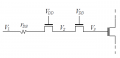# Two NMOS in series (steady state)

#### Koelite

Joined Sep 18, 2023
1
Hello,
I am stuck on an exercise for a while with the circuit as shown below:We have:
$V_{DD}=3.3 V \\ V_{tn0}=0.65 V \\ \gamma=0.5 V^{1/2} \\ 2\phi _{f}=0.6 V \\ V_{1}=2.85 V$

The question is: What is the best approximation for V2 in steady state?
(a) 0 V
(b) 0.65 V
(c) 2.2 V
(d) 2.65 V
(e) 3.3 V

And then same question for V3: What is the best approximation for V3 in steady state?
(a) 0.65 V
(b) 1.55 V
(c) 2.2 V
(d) 2.65 V
(e) 3.3 V

What I could do so far:
We know that the current flowing through the gate of the 3rd transistor is zero, so at least one of the two first NMOS is in cut-off mode or Vds = 0.
We have $V_{GS} = V_G - V_S = V_{DD} - V_2$ and $V_{SB} = V_S - V_B = V_2$.
We also know that $V_{tn} = V_{tn0} + \gamma\left(\sqrt{2\phi_f+V_2}-\sqrt{2\phi_f}\right)$
I tried to compute for each value of V2 possible (from a to e) and the NMOS is cut-off for (d) and (e) V2 = 2.65 V or 3.3 V.
And now I'm stuck, although I know I didn't use the knowledge of the value of V1, or the relation $V_1 - V_D = r_{DS}i_D$.

I would be grateful if you have a hint so I can finish the exercise.

Thank you,
Koelite.

#### WBahn

Joined Mar 31, 2012
29,519
MOD NOTE: Moved to Homework Help.